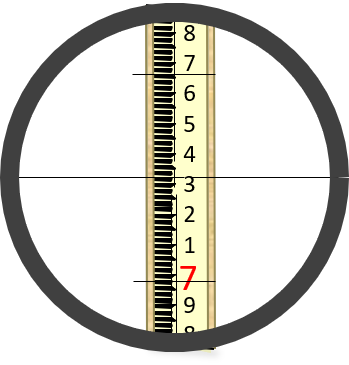## 1. Principle

Stadia is a second tacheometric distance determination method. It has a long history in surveying and was one of the most extensive early topographic mapping methods. A few of the more interesting implementations will be discussed in this chapter to give some historical context. Despite stadia being largely replaced by newer distance measurement technologies, even contemporary instruments still have stadia corosshairs.

The stadia crosshairs, aka stadia wires, are equally spaced above and below the horizontal crosshair on an instrrument's reticule, Figure D-1.Figure D-1Stadia Crosshairs

The line of sight (LoS) of the top hair is the same angle above the central LoS as the lower LoS is below it, Figure D-2.Figure D-2Multiple Lines of Sight

The difference between the top and bottom stadia hair readings on a level rod is the stadia interval, i. The interval in Figure D-3 is (7.66-6.98)=0.68.Figure D-3Stadia Interval

The further away the rod, the greater the interval, Figure D-4.Figure D-4Interval-Distance Relationship

The distance to the rod is the product of the rod interval and the instrument's stadia multiplier, k, Equation D-1.Equation D-1

The multiplier is determined by the stadia and center cross hair spacings.

Older instruments with external focusing telescopes had variable optical geometry and required an additive constant C, Equation D-2.  For most instruments C was 1.Equation D-2

All modern instruments being internal focusing do not need a constant, and most have a multiplier of 100, making the math simple.